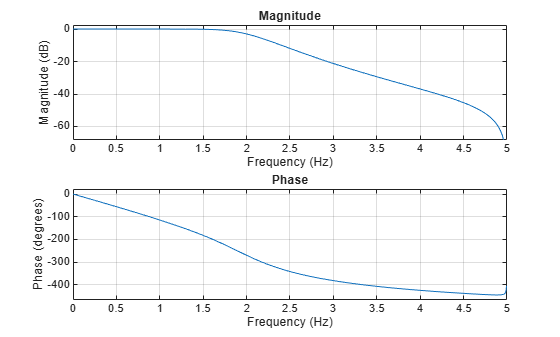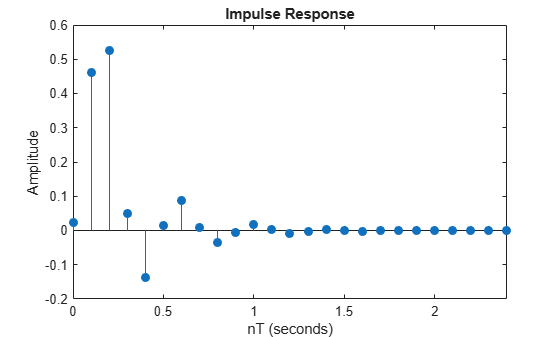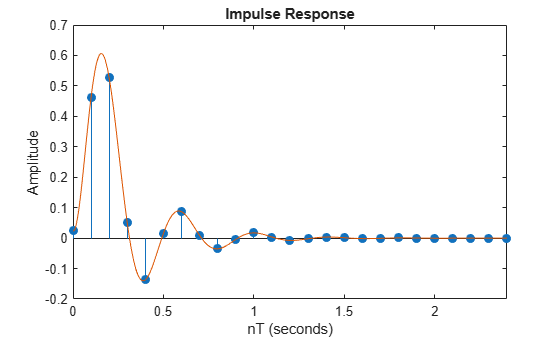# impinvar

Impulse invariance method for analog-to-digital filter conversion

## Syntax

``[bz,az] = impinvar(b,a,fs)``
``[bz,az] = impinvar(b,a,fs,tol)``

## Description

example

````[bz,az] = impinvar(b,a,fs)` creates a digital filter with numerator and denominator coefficients `bz` and `az`, respectively, whose impulse response is equal to the impulse response of the analog filter with coefficients `b` and `a`, scaled by 1/`fs`, where `fs` is the sample rate.```
````[bz,az] = impinvar(b,a,fs,tol)` uses the tolerance specified by `tol` to determine whether poles are repeated.```

## Examples

collapse all

Convert a sixth-order analog Butterworth lowpass filter to a digital filter using impulse invariance. Specify a sample rate of 10 Hz and a cutoff frequency of 2 Hz. Display the frequency response of the filter.

```f = 2; fs = 10; [b,a] = butter(6,2*pi*f,'s'); [bz,az] = impinvar(b,a,fs); freqz(bz,az,1024,fs)```Convert a third-order analog elliptic filter to a digital filter using impulse invariance. Specify a sample rate ${f}_{s}=10$ Hz, a passband edge frequency of 2.5 Hz, a passband ripple of 1 dB, and a stopband attenuation of 60 dB. Display the impulse response of the digital filter.

```fs = 10; [b,a] = ellip(3,1,60,2*pi*2.5,'s'); [bz,az] = impinvar(b,a,fs); impz(bz,az,[],fs)```Derive the impulse response of the analog filter by finding the residues, ${r}_{k}$, and poles, ${p}_{k}$, of the transfer function and inverting the Laplace transform explicitly using

`$H\left(s\right)=\sum _{k}\frac{{r}_{k}}{s-{p}_{k}}⟺h\left(t\right)=\sum _{k}{r}_{k}{e}^{{p}_{k}t}.$`

Overlay the impulse response of the analog filter. Impulse invariance introduces a gain of $1/{f}_{s}$ to the digital filter. Multiply the analog impulse response by this gain to enable meaningful comparison.

```[r,p] = residue(b,a); t = linspace(0,4,1000); h = real(r.'*exp(p.*t)/fs); hold on plot(t,h) hold off```## Input Arguments

collapse all

Analog filter transfer function coefficients, specified as vectors.

Example: `[b,a] = butter(6,2*pi*10,'s')` specifies a 6th-order Butterworth filter with a cutoff frequency of 10 Hz.

Data Types: `single` | `double`

Sample rate, specified as a positive scalar.

Data Types: `single` | `double`

Tolerance, specified as a positive scalar. The tolerance determines whether poles are repeated. A larger tolerance increases the likelihood that `impinvar` interprets closely located poles as multiplicities (repeated ones). The default tolerance corresponds to 0.1% of a pole magnitude. The accuracy of the pole values is still limited to the accuracy obtainable by the `roots` function.

Data Types: `single` | `double`

## Output Arguments

collapse all

Digital filter transfer function coefficients, returned as vectors.

## Algorithms

`impinvar` performs the impulse-invariant method of analog-to-digital transfer function conversion discussed in reference :

1. It finds the partial fraction expansion of the system represented by `b` and `a`.

2. It replaces the poles `p` by the poles `exp(p/fs)`.

3. It finds the transfer function coefficients of the system from the residues from step 1 and the poles from step 2.

 Antoniou, Andreas. Digital Filters. New York: McGraw-Hill, Inc., 1993.

 Parks, Thomas W., and C. Sidney Burrus. Digital Filter Design. New York: John Wiley & Sons, 1987.